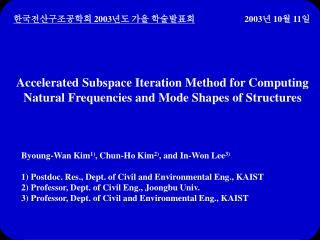DownloadDownload PresentationAccelerated Subspace Iteration Method for Computing

# Accelerated Subspace Iteration Method for Computing

Télécharger la présentation## Accelerated Subspace Iteration Method for Computing

- - - - - - - - - - - - - - - - - - - - - - - - - - - E N D - - - - - - - - - - - - - - - - - - - - - - - - - - -
##### Presentation Transcript

1. 한국전산구조공학회 2003년도 가을 학술발표회 2003년 10월 11일 Accelerated Subspace Iteration Method for Computing Natural Frequencies and Mode Shapes of Structures Byoung-Wan Kim1), Chun-Ho Kim2), and In-Won Lee3) 1) Postdoc. Res., Dept. of Civil and Environmental Eng., KAIST 2) Professor, Dept. of Civil Eng., Joongbu Univ. 3) Professor, Dept. of Civil and Environmental Eng., KAIST

2. Contents • Introduction • Proposed method • Numerical examples • Conclusions

3. Introduction • Background • Dynamic analysis or seismic design of structures •  Natural frequencies and mode shapes •  Eigenvalue analysis • Eigensolution methods • - Subspace iteration method (Bathe & Wilson, 1972) • - Lanczos method (Lanczos, 1950) • Subspace iteration method • - Widely used in structural problems • - Various improved versions are developed. • - Subspace iteration method with Lanczos starting subspace • (Bathe & Ramaswamy, 1980)

4. In quantum problems, Grosso et al. (1993) proposed • accelerated Lanczos recursion with squared operator. a, b = coefficients f = basis functions for quantum systems H = operator Et = trial energy

5. Objective • To improve the subspace iteration method with • Lanczos starting subspace by applying the square technique to • generation of Lanczos vectors used as starting vectors

6. Proposed method • Subspace iteration method with conventional Lanczos starting subspace • Eigenproblem of structures mass matrix (n n) stiffness matrix (n n) eigenvectors set (n q) diagonal matrix with eigenvalues (q  q) n = system order q = 2p p = no. of desired eigenpairs

7. Generation of starting vectors by Lanczos algorithm

8. Simultaneous inverse itertion and system reduction • Eigensolution for reduced system and eigenvector calculation

9. Subspace iteration method with proposed Lanczos starting subspace • Generation of starting vectors by proposed Lanczos algorithm

10. Squared dynamic matrix can separate Riz values more rapidly. •  Starting vectors are closer to exact eigenvector space. •  The number of iterations is reduced. • Simultaneous inverse itertion, system reduction, • eigensolution for reduced system and eigenvector calculation • are the same as the conventional method.

11. Numerical examples • Structures • Building structure with 1008 DOFs • Building structure with 5040 DOFs • Error measure for checking convergence

12. Building structure with 1008 DOFs • Geometry and material properties A = 0.01 m2 I = 8.310-6m4 E = 2.11011Pa  = 7850 kg/m3

13. Number of iterations

14. Computing time hj = modal contribution factors s = spatial load distribution vector

15. Building structure with 5040 DOFs • Geometry and material properties A = 0.01 m2 I = 8.310-6m4 E = 2.11011Pa  = 7850 kg/m3

16. Number of iterations

17. Computing time

18. Conclusions • Subspace iteration method with proposed Lanczos starting subspace has smaller number of iterations than the subspace iteration method with conventional Lanczos starting subspace because squared dynamic matrix in proposed algorithm can accelerate convergence. • Since proposed method has less computing time than the conventional method when the number of desired eigenpairs is small, proposed method is practically efficient.